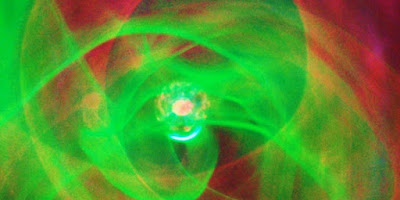# Time is Changing as Space ChangesPlasma Ball Close-Up shot with Open Shutter.(Taken by me)

Of what we constantly try to achieve in the physics of science, is continuity and defined systems. By now, most physicist know that in the world of quantum, the approach is by the study of the many and cannot be by the study of a single particle / case.

The first and simple example of the quantum physics is how nuclear decay is calculated for every decaying material. The half-life is estimated for a very large sum of atoms, but the half-life of an individual atom can never be calculated.

The equation of decay is based on the following form :

λ is the decay constant which is different for every radioactive material. The expected decay −dN/N (decaying atoms per atoms present) is proportional to an increment of time, dt.
So far, what is explained above is nothing new to the scientific approach, but it is worth mentioning that time which is considered a constant measurable value, is in fact a varying value. In Einstein’s theory of relativity, time has a changing value if the measuring device is in motion, time is also varying in the presence gravitational fields.
The method with which we measure the age of things for geology and archaeology is based on nuclear decay. If we assume “t” has always been of exact value, then every dating is considered accurate.
If we considered that “t” has varied, maybe increased, then all what we know about the time scales of change and the universe, could be wrong.
There are theories that the universe is expanding faster and faster, which would mean that the time constant is changing. At least the Universe has definitely expanded since the Big Bang till today. Again if the universe is expanding then all gravitational fields in the universe are weakening as the large masses are moving away from each other in a general definition and then time is shortening.  Based on that knowledge we could theories that time is not a constant across time and thus we must rethink how we view time.
It is possible, in an exaggerated manner that at a point in the past the 1 second we measure today used to be 0.5 seconds and thus in today’s constant the nuclear decay is two times faster. Anything we date today as 10,000 year old, could be on today’s scale of time 5000 year old.
As we start to think about time as a varying value, and as we know that relative speed and gravitational fields, have effects on that, we could also conclude that time is varying in flow across the distances of space. Now, we do observe stars from far distances and Nebulae, we analyze their chemical composition by several methods, one of them is the light spectrum and the Doppler shift we use to measure their relative speed. Now if we are measuring speed based on a constant time, or based on a light frequency which itself is based on time; what if time varies across space. Then all measurements are faulty and it would be really hard to correct as time would then need to be measured in all areas that light travels in to reach us.
There are few assumptions we could also generate:
1)The age of earth could be wrong and same for the sun.
2)The universe could be expanding at places, and contracting at others. There is a chance that the entire universe is in an oscillation phase which could lead back to the one dot universe and repeat the big bang or could oscillate in around median size infinitely.
3)There is no evidence that the world few thousands year ago, used to be like we observe it today. We could be passing through a phase of relative stagnation at the top of the sinusoidal oscillation curve or maybe at the bottom. The scale of space evolution relative to time is far larger than the scale of days or years we could measure. If the nature of the universe changes every 1 billion years and we are in the middle of that phase, there is no way we could measure it. The counterargument would be , but we can observe stars and nebulas dating billions of years. My answer billions of years on the scale of time we use on earth today. This scale of time again might change across the universe between us and that star.
4)Materials that we thought stars or nebula gas are made of, could be different from the light spectrum we received.Assuming photon oscillation frequencies could be changing across space where time is different.
One final thought : As time slows down at speeds close to the speed of light, then would it stop at the speed of light ? The Energy of a photon cannot be dissipated if it is trapped in time. Only an interaction with other particles can release the energy of a photon.

Scroll to top